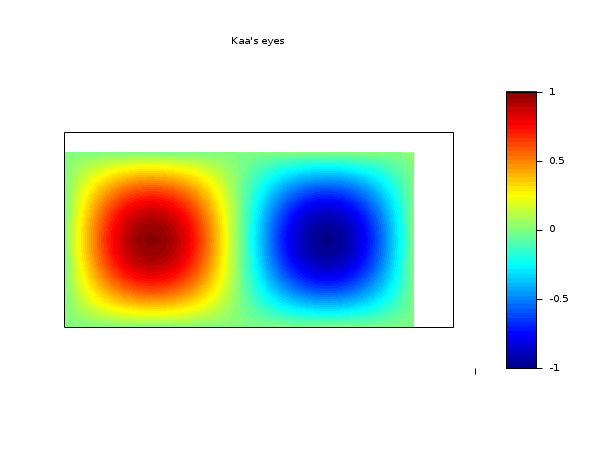Scilab Home page | Wiki | Bug tracker | Forge | Mailing list archives | ATOMS | File exchange
Change language to: English - Français - Português - 日本語 -

Please note that the recommended version of Scilab is 6.0.2. This page might be outdated.
See the recommended documentation of this function

Справка Scilab >> Графики > 2d_plot > Sgrayplot

# Sgrayplot

smooth 2D plot of a surface using colors

### Calling Sequence

```Sgrayplot(x,y,z,<opt_args>)
Sgrayplot(x,y,z [,strf, rect, nax, zminmax, colminmax, mesh, colout])```

### Arguments

x,y

real row vectors of size n1 and n2.

z

real matrix of size (n1,n2). `z(i,j)` is the value of the surface at the point (x(i),y(j)).

<opt_args>

This represents a sequence of statements `key1=value1,key2=value2`,... where `key1`, `key2,...` can be one of the following: strf, rect, nax, zminmax, colminmax, mesh, colout.

strf

is a string of length 3 `"xyz"` (by default `strf= "081"`)

x

controls the display of captions.

x=0

no caption.

x=1

captions are displayed. They are given by the optional argument `leg`.

y

controls the computation of the actual coordinate ranges from the minimal requested values. Actual ranges can be larger than minimal requirements.

y=0

no computation, the plot use the previus (or default) scale

y=1

from the rect arg

y=2

from the min/max of the x, y data

y=3

built for an isometric scale from the rect arg

y=4

built for an isometric plot from the min/max of the x, y data

y=5

enlarged for pretty axes from the rect arg

y=6

enlarged for pretty axes from the min/max of the x, y data

y=7

like y=1 but the previus plot(s) are redrawn to use the new scale

y=8

like y=2 but the previus plot(s) are redrawn to use the new scale

z

controls the display of information on the frame around the plot. If axes are requested, the number of tics can be specified by the `nax` optional argument.

z=0

nothing is drawn around the plot.

z=1

axes are drawn, the y=axis is displayed on the left.

z=2

the plot is surrounded by a box without tics.

z=3

axes are drawn, the y=axis is displayed on the right.

z=4

axes are drawn centred in the middle of the frame box, with the box disabled.

z=5

axes are drawn centred in the middle of the frame box, with the box enabled.

rect

This argument is used when the second character y of argument `strf` is 1, 3 or 5. It is a row vector of size 4 and gives the dimension of the frame: `rect=[xmin,ymin,xmax,ymax]`.

nax

This argument is used when the third character z of argument `strf` is 1. It is a row vector with four entries `[nx,Nx,ny,Ny]` where `nx` (`ny`) is the number of subgraduations on the x (y) axis and `Nx` (`Ny`) is the number of graduations on the x (y) axis.

zminmax, colminmax, mesh, colout

See fec.

### Description

`Sgrayplot` is the same as `grayplot` but the plot is smoothed. The function `fec` is used for smoothing. The surface is plotted assuming that it is linear on a set of triangles built from the grid (here with n1=5, n2=3):

```_____________
| /| /| /| /|
|/_|/_|/_|/_|
| /| /| /| /|
|/_|/_|/_|/_|```

The function colorbar may be used to see the color scale.

The parameter `zminmax` is useful for animation purpose (see an example after) and the parameter `colminmax` lets the user choose a part of the current colormap (see the fec help page).

Enter the command `Sgrayplot()` to see a demo.

### Examples

```// example #1
x=-10:10; y=-10:10;m =rand(21,21);
clf()
xset("colormap",hotcolormap(64))
Sgrayplot(x,y,m, strf="011", rect=[-20,-20,20,20])``````// example #2
t=-%pi:0.1:%pi; m=sin(t)'*cos(t);
clf()
xset("colormap",jetcolormap(64))
colorbar(-1,1)
Sgrayplot(t,t,m, strf="041")``````// example #3: an animation display cos(t)*sin(x)sin(y).
n = 30;
nt = 100;
x = linspace(0,2*%pi,n);
y = linspace(0,%pi,n/2);
z = sin(x')*sin(y);
t = linspace(0,4*%pi,nt);
show_window(); clf()
f=gcf();
f.color_map=jetcolormap(64);
colorbar(-1,1)
Sgrayplot(x,y,cos(t(1))*z, strf="042", zminmax=[-1,1])
c=gce(),e=c.children
xtitle("Kaa''s eyes")
for i = 1:nt
e.data(:,3)=matrix(cos(t(i))*z,-1,1);
end```• fec — pseudo-color plot of a function defined on a triangular mesh
• fgrayplot — 2D plot of a surface defined by a function using colors
• grayplot — 2D plot of a surface using colors
• Sfgrayplot — сглаженный двумерный график поверхности, определённой через функцию, с использованием цветов
• colorbar — draw a colorbar